# Conditional Probability – Law of Total Probability

In this lesson, we would examine Law of Total Probability. We looked Multiplication Law previously.

I would state this law. Then give an explanation. Finally we would apply it to solve some problems. We would not worry about proving it as this is not necessary.  At least, for now.

The Law of Total Probability states that:

Let B1, B2, …, Bn be such that: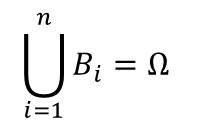and Bi  Bj = ø for all i  ≠ j, with P(Bi) > 0 for all i.

Then for any event A,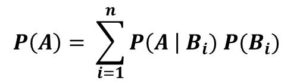Explanation

The events Bi are mutually disjoint events whose union is Ω. Then to find the probability of an event A, we take the sum of all the conditional probabilities of A given Bi. This would be taken over Bi.

Let’s now take some examples to see how it applies.

#### Example 1 (Taken from previous lesson)

A bag contains 3 red balls and 1 blue ball.

Two balls are selected without replacement. What would be the probability that the second ball selected is red?

Now we can apply the Law of Universal Probability

To find the probability that the second ball is red, we need to sum two probabilities:

• Probability that the second ball is red given that the first ball is rd
• Probability that the second ball is red given that the first ball is blue

Let P(R1) be the probability that the first ball is red

P(R2) be the probability that the second ball is red

P(B1) be the probability that the first ball is blue

P(R2) = P(R2 | R1) P(R1) + P(R2 | B1) P(B1)

= (2/3) * (3/4) + 1 * (1/4) = 3/4

Let’s take another example

#### Example 2

Assuming  that occupations are grouped into three levels: Upper(U), Middle(M) and Lower(L) levels.

U1 denotes the event that a father’s occupation is upper level

U2 denotes that the son’s occupation is upper level

M1 denotes that the father’s occupation is upper level and so on.

This information is represented in the table below for a particular city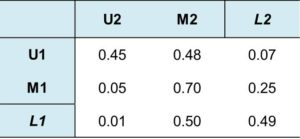This table is called matrix of transition probabilities. It gives value of conditional probabilities

Assuming that from the father’s generation, 10% are in U, 40% are in M and 50% are in L. What is the probability that the son (next generation) is in U?

For the value in row U1 and column U2, we say ‘if the father is in U, then the probability that the son is in U is 0.45.

Therefore, P(U2 | U1) = 0.45

Also, P(M2, | U1) = 0.48 and so on.

For the value in row U1 and column M2, we say ‘if the father is in U, then the probability that the son is in M is 0.48

Let’s now apply the Law of Universal Probability to solve the problem.

The probability that the son (next generation) is in U is given by the summation of 3 conditional probabilities

• probability that the son is in U given that the father is in U  which is  P(U2 | U1) P(U1)
• probability that the son is in U given that the father is in M   which is  P(U2 | M1) P(M1)
• probability that the son is in U given that the father is in L  which is  P(U2 | L1) P(L1)

Therefore:

P(U2)P(U2 | U1) P(U1) + P(U2 | M1) P(M1) + P(U2 | L1) P(L1)

= 0.45 x 0.1 + 0.05 x 0.4 + 0.01 x 0.5

= 0.07

In the same way, P(M2) and P(L2) can also be calculated. I give that to you as an assignment. Leave your answer in the comment box below.

#### Example 3

Still based on the matrix of transition probabilities in Example 2. Support the son is in level U2, what is the probability that his father is in level U1.

In this case, we are given the output  probability and need to determine the conditional probabilities. So it’s like we need to work backwards.

To solve this, simple need to rearrange the Multiplication Law Formula.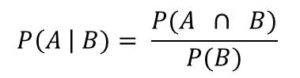Applying it to the problem on ground, we have: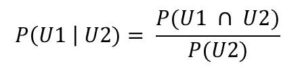But we know that:

P(U1 ∩ U2) = P(U2 | U1) P(U1)

and from law of total probability in example 3, we have:

P(U2) = P(U2 | U1) P(U1) + P(U2 | M1) P(M1) + P(U2 | L1) P(L1)

Therefore we now have: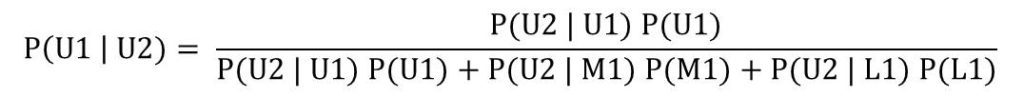The value of the numerator is 0.45 x 0.1 = 0.045

Value of the denominator is 0.07. Calculated from Example 2

Therefore P(U1 | U2) = 0.045/ 0.07 = 0.64

In other words, 64% of the children in upper level occupations (U2) have there fathers in level U2 as well

Now we have touched on Bayes’ Rule. We would now examine Bayes’ Rule in more detail in the next lesson.#### kindsonthegenius

Kindson Munonye is currently completing his doctoral program in Software Engineering in Budapest University of Technology and Economics

View all posts by kindsonthegenius →